Symmetry

Chapter 13 Class 6 Symmetry
Concept wise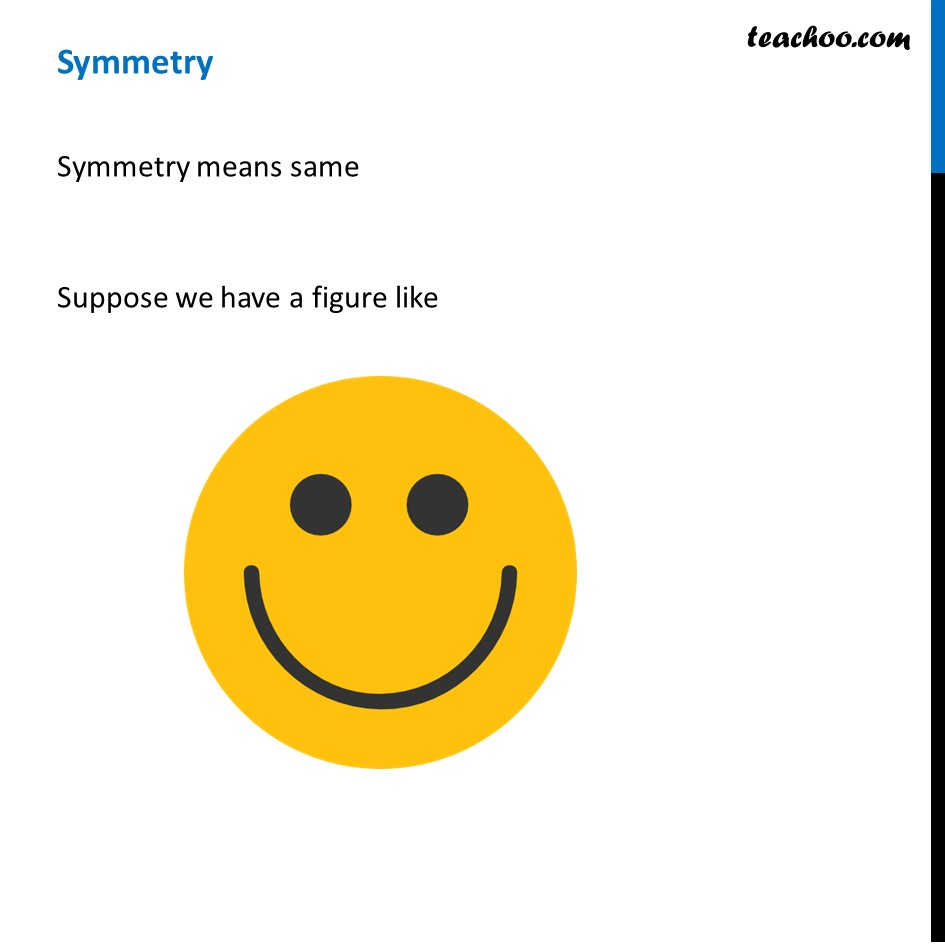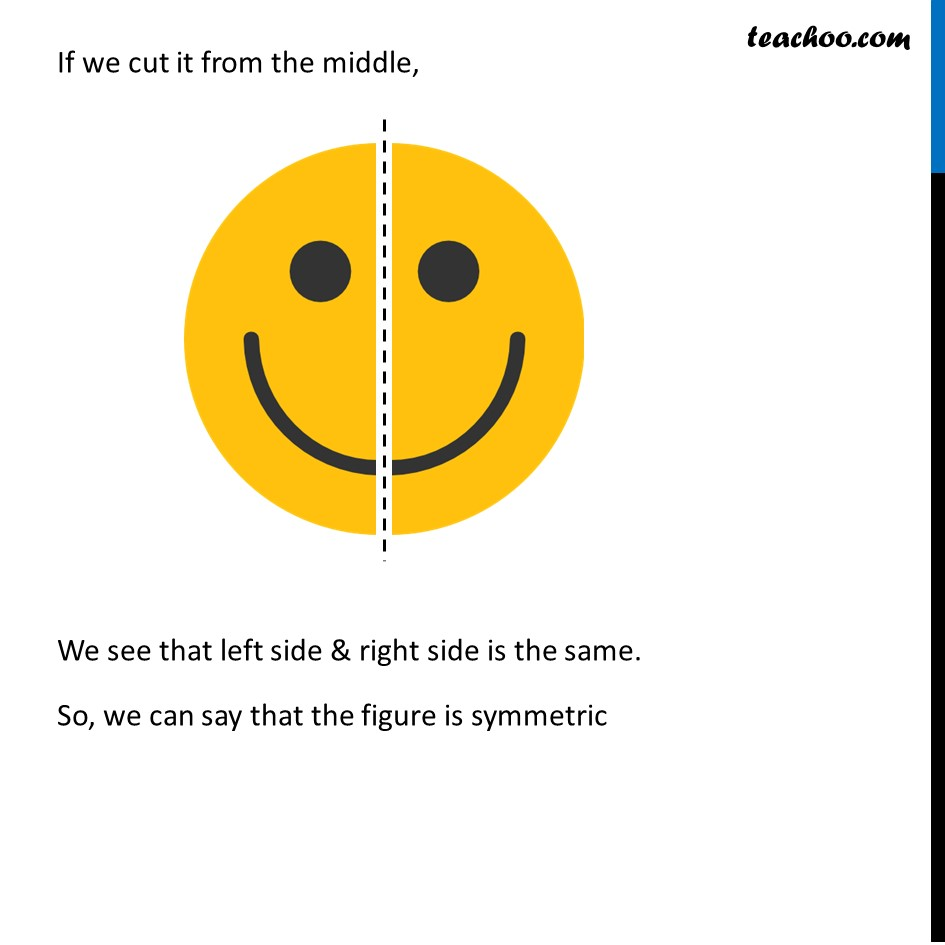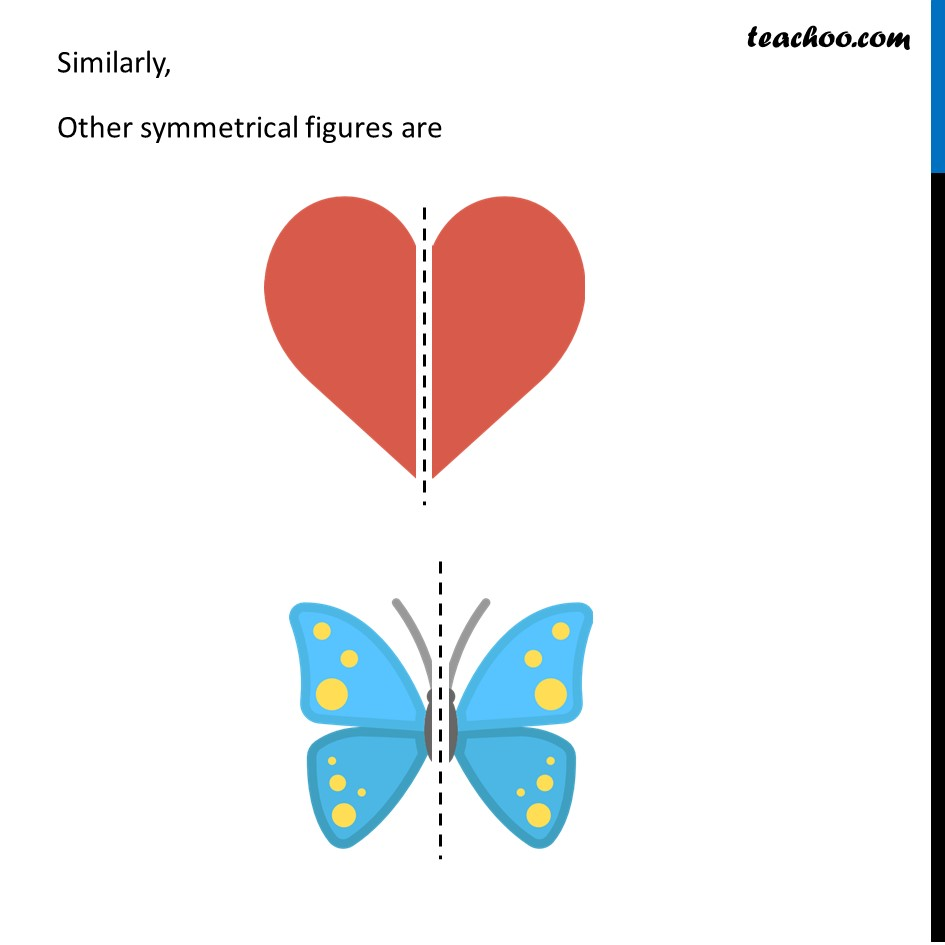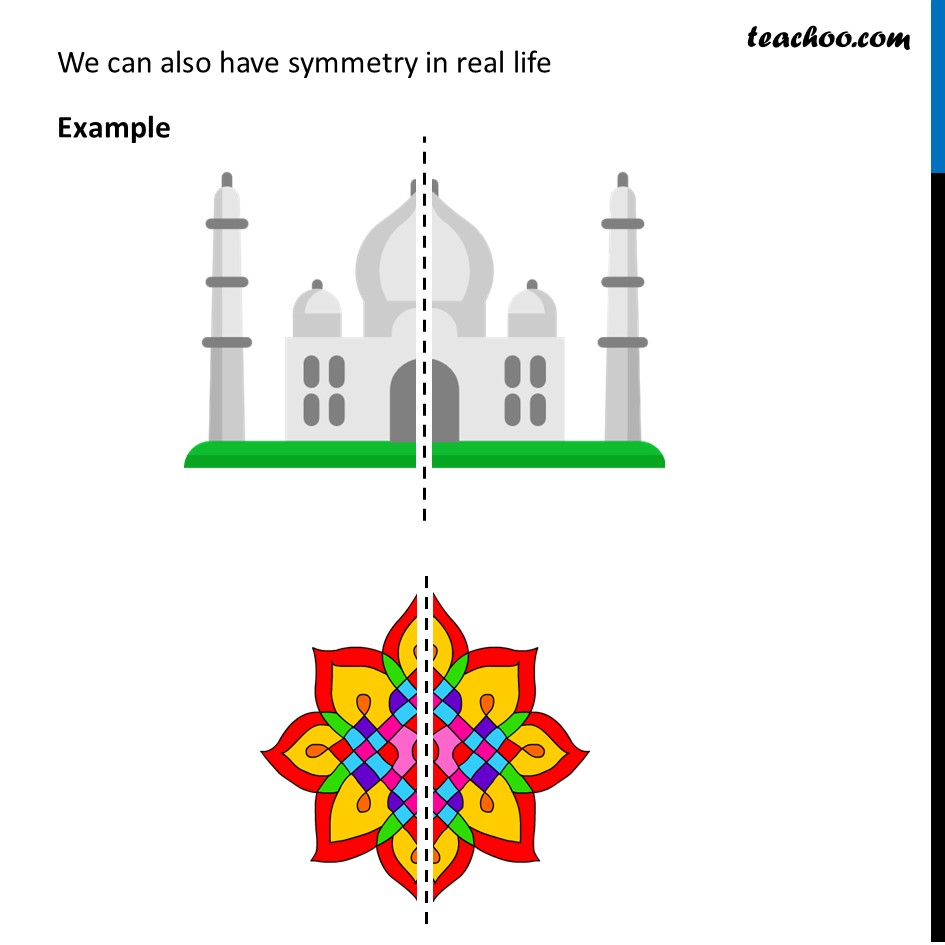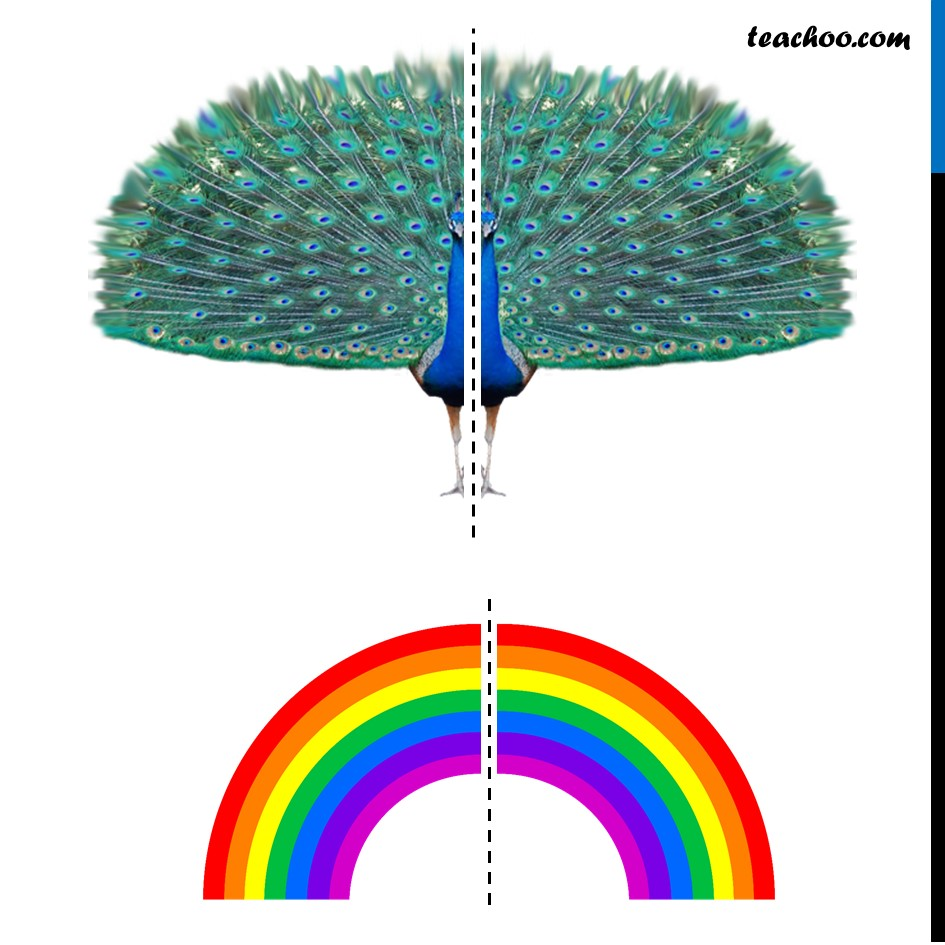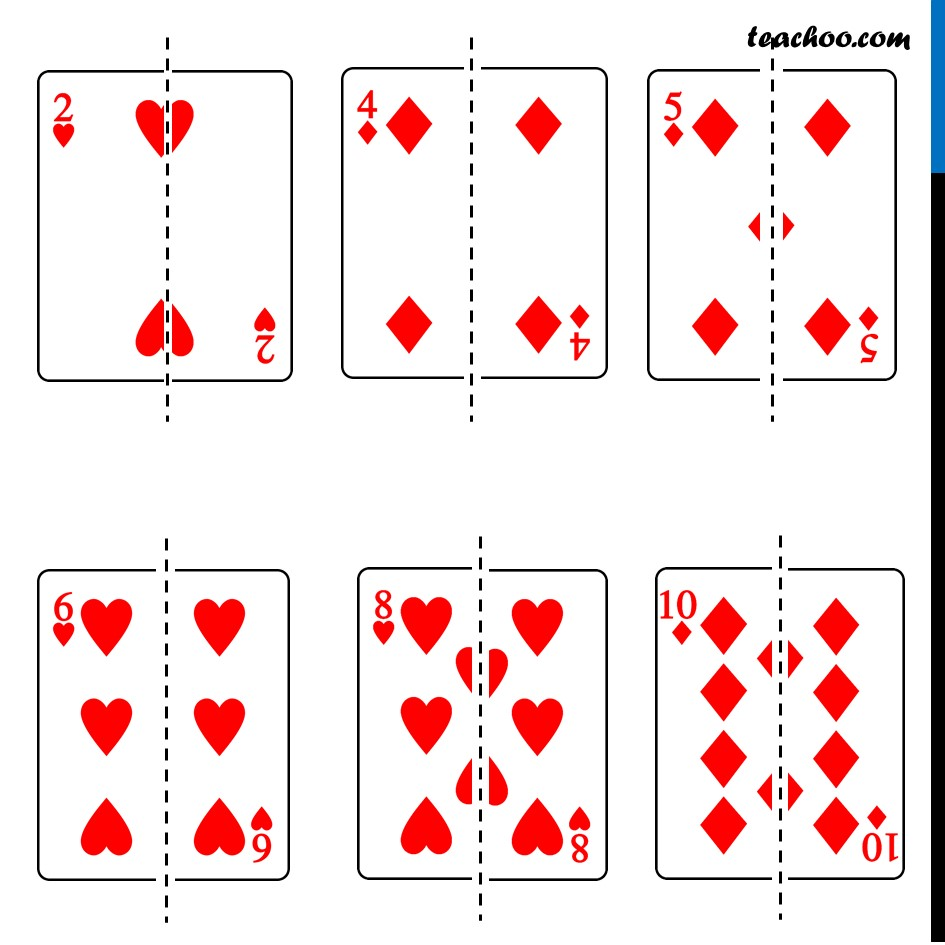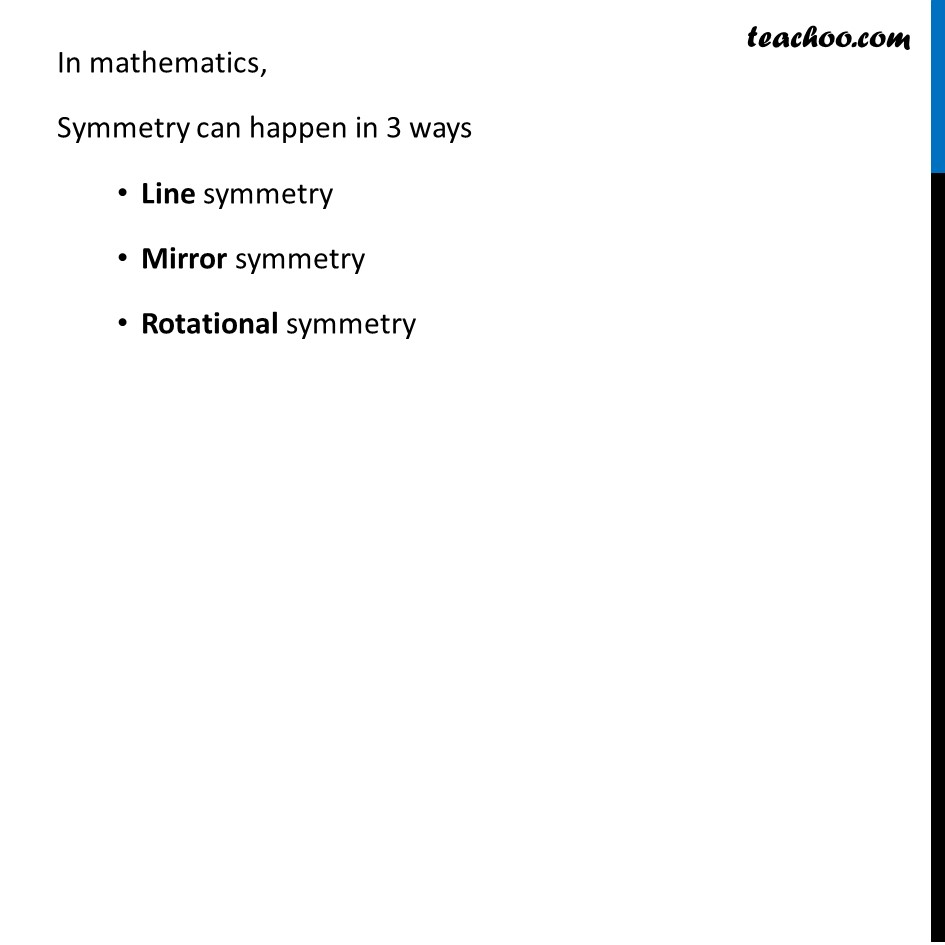### Transcript

SymmetrySymmetry means same Suppose we have a figure like If we cut it from the middle, We see that left side & right side is the same. So, we can say that the figure is symmetric If we cut it from the middle, We see that left side & right side is the same. So, we can say that the figure is symmetric Similarly, Other symmetrical figures are We can also have symmetry in real life Example In mathematics, Symmetry can happen in 3 ways Line symmetry Mirror symmetry Rotational symmetry